He (Dase) multiplied together mentally two 8-figure numbers in 54 seconds, two 20-figure numbers in 6 minutes, two 40-figure numbers in 40 minutes, and two 100-figure numbers in 8 hours; he could extract the square root of a 60-figure number in an "incredibly short time", and the square root of a 100-figure number in 52 minutes.

Johann Dase (1824-1861) was born in Hamburg and began public exhibitions of his talents when he was 15. Dase could also count objects with the greatest rapidity. With a single glance he could give the number (up to thirty or thereabouts) of peas in a handful scattered on a table; and the ease and speed with which he could count the number of sheep in a herd, of books in a case, or the like, never failed to amaze the beholder.

Chapter 6 (Part 1)

Vertically and Crosswise

We come now to the general method of multiplication by which any two numbers, no matter how long, can be multiplied together by means of a simple pattern.

We will see a number of variations of this including squaring and the reverse process of division.

General Multiplication

This Vertically and Crosswise formula develops the left to right method of multiplication described in chapter 1 for multiplication by a single figure, and is surely the most efficient general method of multiplication possible,

In this chapter we will put the numbers being multiplied one below the other.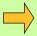Example 1There are 3 steps:
A. multiply vertically in the left-hand
column: 2 × 2 = 4,
so 4 is the first figure of the answer.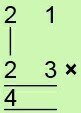B. multiply crosswise and add:
2 × 3 = 6,
1 × 2 = 2, 6 + 2 = 8,
so 8 is the middle figure of the answer.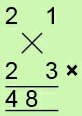C. multiply vertically in the right-hand
column: 1 × 3 = 3,
3 is the last figure of the answer.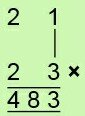Example 2A. vertically on the left: 1 × 2 = 2,
B. crosswise: 1 × 1 = 1, 4 × 2 = 8 and 1 + 8 = 9,
C. vertically on the right: 4 × 1 = 4.

Question Comment !
 Tutorial Title Question Title No Questions 0 Questions Asked 0 Skipped Questions 0 Correctly Answered 0 Wrongly Answered 0 Total Question Attempts 0 Current Question Attempts 0

Skip Question

Reset Test

Halt Test

 No Questions 0 Questions Asked 0 Skipped Questions 0 Correctly Answered 0 Wrongly Answered 0 Total Question Attempts 0

Time Taken

CloseExercise A

The previous examples involved no carry figures, so let us consider this next.Example 3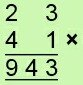The 3 steps give us:

2 × 4 = 8,
2 × 1 + 3 × 4 = 12,
3 × 1 = 3.

In building up the answer mentally from the left we combine these numbers as we did in Chapter 1.

The mental steps are:

8
8,14 = 94 (the 1 is carried over to the left)
94,3 = 943Example 4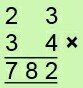The steps are:

6
6,17 = 77
77,12 = 782Example 5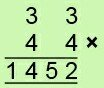The steps are:

12
12,24 = 144
144,12 = 1452

We can now multiply any two 2-figure numbers together in one line. With practice we will find that we can multiply the numbers mentally.Exercise B

You may have found in this exercise that you prefer to start with the crosswise multiplications, and put the left and right vertical multiplications on afterwards.

This method also works perfectly for algebraic multiplications (and divisions).

Explanation  It is easy to understand how this method works. The vertical product on the right multiplies units by units and so gives the number of units in the answer. The crosswise operation multiplies tens by units and units by tens and so gives the number of tens in the answer. And the vertical product on the left multiplies tens by tens and gives the number of hundreds in the answer.

Challenge Question: Can you see how this method simplifies when

1. both numbers end in a 1
2. the last figures of the numbers, or the first figures, or both figures of one number, are the same?

Using The Vinculum

We now consider again the helpful vinculum. This was described in the last chapter and simplifies calculations by removing large digits like 6, 7, 8 and 9.Example 6

29 × 34: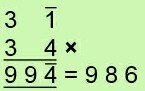We write 29 as 31 in order to remove the large digit 9.
Then the 3 steps are: 3 × 3 = 9, 3 × 4 + 1 × 3 = 12 - 3 = 9, 1 × 4 = 4.
The 4 may then be removed as shown in the previous chapter (994 = 990 - 4 = 986).

Of course the use of the vinculum is optional but it does remove the large digits, and the plus and minus numbers tend to cancel each other out.Example 7

49 × 58: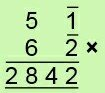The mental steps are:

30
30,-16 = 316 = 284
284,2 = 2842Example 8

28 × 42:The mental steps are:

12
12,2 = 122
122,4, = 1224 = 1176

At which point to remove the vinculums is a matter of judgment, the answer being the same of course whatever method is decided upon.Exercise C

Challenge Question: Can you prove the By One More than the One Before method from Chapter 3 for finding products like 34 × 36 and 452, using Vertically and Crosswise?